﻿ Heat Transfer Enhancement in an Axially Rotating Pipe with Twisted Tape InsertPublications are Open
Access in this journal
Article Versions
Export Article
• Normal Style
• MLA Style
• APA Style
• Chicago Style
Research Article
Open Access Peer-reviewed

### Heat Transfer Enhancement in an Axially Rotating Pipe with Twisted Tape Insert

Obed Y.W. Abotsi , J.P. Kizito
American Journal of Mechanical Engineering. 2020, 8(1), 1-8. DOI: 10.12691/ajme-8-1-1
Received December 15, 2019; Revised January 20, 2020; Accepted January 28, 2020

### Abstract

This paper addresses heat transfer enhancement in an axially rotating pipe under constant wall heat flux condition. Numerical simulations were processed using the Reynolds Average Navier-Stoke (RANS) solver of ANSYS Fluent 19.2. The realizable k-ε turbulence model was used to provide closure to the RANS equations. Buoyancy effects (β∆T) and gravity (g) were considered in the numerical analysis. Parametric runs were made for axial Reynolds numbers between 10000 and 30000 (10000 ≤ Rea ≤ 30000) at various rotation rates (0 ≤ N ≤ 3) using water as the working fluid. CFD results showed that increasing the rotation rate (N) augmented the Nusselt number. Nusselt number augmentation was associated to increase in the strength of secondary flow. The influence of twisted tape insert on the thermal performance of the rotating pipe was also investigated. Twisted tape produced additional swirl to further enhance the heat transfer rate. The intensity of swirl created by the inserted tape depended on the twist ratio. Three different twist ratios (P/W) of 3, 5 and 7 (P is the pitch and W is the width of the tape) were examined. Simulation results revealed that decreasing the twist ratio of the tape augmented the heat transfer rate.

### 1. Introduction

Flow through axially rotating pipes have numerous engineering applications such as flow in power transmission shafts, rotors, combustion chambers, turbomachines, heat exchangers, cyclone separators and nuclear reactors 1, 2, 3, 4, 5. Many theoretical, numerical and experimental investigations have been carried out by researchers to study the effect of pipe rotation on the flow structure and heat transfer.

When fluid enters an axially rotating pipe, tangential forces which occurs between the walls of the rotating pipe and the fluid cause the fluid to rotate with the pipe resulting in a flow pattern which is different from that noticed in a stationary pipe 1. Thus, equations governing flow through stationary pipes cannot be employed in analyzing flow through rotating pipes.

For turbulent flows in rotating pipes, Borisenko et al. measured the effect of rotation on the turbulence fluctuations by using hot wire probes 6. Their paper showed that rotation suppresses turbulence fluctuations and when the flow advanced downstream, the suppressions extended towards the central part of the pipe. Pedley revealed that a Hagen-Poiseuille flow is unstable to infinitesimal disturbance when forced to rotate about its axis 7. Pedley also analyzed the stability of fully developed laminar flow in a rotating pipe 8. He showed that for solid body rotation, flow instabilities began when the axial Reynolds number exceeded 82.9. Experimental studies performed by Nagib et al. with a hot thermistor anemometer and flow visualization techniques confirmed these results 9. By measuring the velocity profile across the pipe’s cross-sections at various axial positions from the entrance, Murakami and Kikuyama examined the flow pattern in an axially rotating pipe when a fully developed turbulent flow was introduced 10. They observed that with an increase in pipe rotational speed, the axial velocity profile approaches the Hagen-Poiseuille flow.

Kikuyama et al. measured the mean velocities and turbulent fluctuations inside the developing turbulent boundary layer of a rotating pipe by using two types of hot-wire probes 11. Experimental results revealed that rotation has two opposite effects on the flow: a destabilizing effect due to large shear near the inlet area of the rotating pipe wall, and a stabilizing effect caused by the centripetal force of the swirling velocity component which becomes dominant in the downstream region. The turbulence fluctuations observed in the rotating pipe was lower than in a stationary pipe. Imao et al, studied the turbulent characteristics of a fully developed flow in an axially rotating using a single-component Laser Doppler Velocimetry 12. They revealed that, turbulent fluctuations and the rate of turbulence energy transport decrease with an increase in the rotating speed of the pipe. Kikuyama et al. investigated the velocity distribution and friction coefficient in the fully developed region of a pipe rotating about its longitudinal axis 13. They used the correlation between the mixing length and Richardson number proposed by Bradshaw 14 for theoretical analysis and verified their results with experiments. They observed that the flow pattern changed according to the rotation rate of the pipe. Rotation rate is defined as the ratio of the rotational Reynolds number to the axial Reynolds number. From their results, they concluded that increasing the rotation rate laminarizes an initially turbulent flow (cause an already turbulent flow to reverse back to a laminar flow) and destabilizes an initially laminar flow.

In their paper, Seghir-Ouali et al. studied heat transfer in a rotating cylinder with air as working fluid at various pipe rotational speeds (4 to 880 rpm) 15. They showed that increasing the rotational speed of the pipe increased the convective heat transfer coefficient. Gai et al. passed 50-50 ethylene glycol-water mixture through a hollow shaft with 1 kw heat load and observed the thermal performance at different rotational speeds 16. CFD results revealed that increasing pipe rotating speed significantly increased the convective heat transfer of the shaft above the stationary condition. Chong determined the pressure loss across the rotor-stator gap by using both experimental and numerical approaches 17. With air as the cooling fluid, he showed that rotation introduces rotational pressure losses and proposed a correlation for calculating the total pressure loss in a rotating systems. For certain combinations of axial and rotational Reynolds numbers, the rotational pressure loss contributed to about 50% of the total system loss. Authors 18, 19 and 20 also indicated that rotation improves heat transfer. However, there is limited literature on heat transfer augmentation at a given rotating speed.

Twisted tapes are used for heat transfer enhancement in stationary pipes/systems due to their effectiveness, low cost and ease of installation 21. Heat transfer augmentation by the twisted tape is attributed to swirling flows which promotes fluid mixing and disrupts the thermal boundary layer. An extensive survey of theoretical, experimental and numerical literature related to twisted tapes have been carried out by Dewan et al 22. Most of these studies examined the influence of twisted tape on the heat transfer, the hydraulic loss and the flow pattern. Koch 23, Kreith and Margolis 24, Seigel 25 and Date 26, investigated turbulent flow of water through a pipe equipped with twisted tape. Hong and Bergles 27, Wang and Sunden 28, and Lin and Wang 29 studied laminar water flow through a tube with twisted tape. The general conclusion from these studies 23, 24, 25, 26, 27, 28, 29 is that twisted tapes improves the heat transfer coefficient. The corresponding pressure drop associated with tubes equipped with twisted tape were much higher than in plain tubes.

The objective of this study is to investigate the influence of twisted tape inserts on the flow structure and heat transfer of an axially rotating pipe. The dimensionless form of the governing equations and numerical procedures are presented in the next section. The effect of varying the twist ratio of the inserted tape on the thermal and hydraulic performance of the rotating pipe is discussed.

### 2. Mathematical Model

The analysis of flow in axially rotating pipes vary greatly from stationary pipes due to the presence of secondary flow. As fluid runs through the rotating pipe, a density gradient (temperature induced variation of the fluid density) is formed. This causes the relatively colder and denser fluid particles at the center to move outwards and for the warmer and less dense fluid particles at the pipe wall to move inwards. Interactions between centripetal and coriolis acceleration components together with the density gradient generates a complex secondary flow. The regular flow combines with the secondary flow to produces a helical fluid pathway.

• Figure 1. Schematic of an axially rotating pipe with heat flux

Fluid flow in an axially rotating pipe is governed by the unsteady incompressible Navier-Stokes equation. Figure 1 depicts a straight horizontal pipe rotating about its own axis with constant angular velocity, Ω. The radial, azimuthal and axial velocity components corresponding to the directions r, θ and z, are given as Vr, Vθ and Vz respectively. The imposed wall heat flux, q”, is assumed to be uniform and constant. The equations of continuity, momentum and energy are presented below:

Continuity Equation: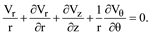(1)

Momentum Equation:

r component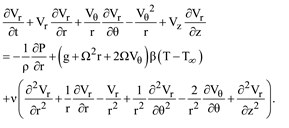(2)

θ component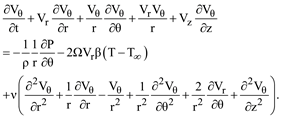(3)

z component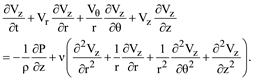(4)

Energy Equation:(5)

where ϕ, the viscous dissipation rate is:(6)

The dimensionless variable used are:The dimensionless form of the momentum and energy equations are presented below:

Momentum Equation:

r component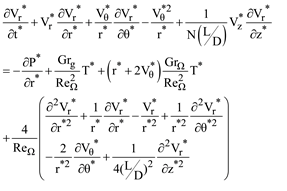(7)

θ component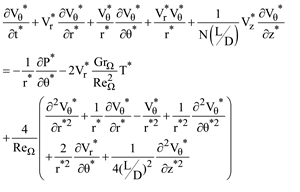(8)

z component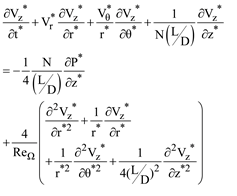(9)

Energy Equation: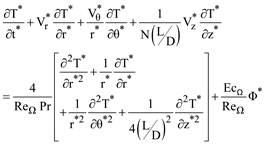(10)

where ϕ*, the dimensionless rate of viscous dissipation is: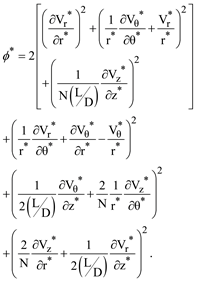(11)

From the above equations, it can be inferred that flow through an axially rotating pipe is influenced by the following parameters: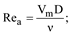axial Reynolds number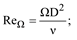rotational Reynolds number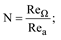rotation rate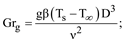gravitational Grashof numberrotational Grashof numberPrandtl number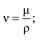kinematic viscosity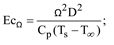Eckert numberAspect ratio

where ρ, μ, k, β and Cp are the density, dynamic viscosity, thermal conductivity, coefficient of thermal expansion and the specific heat capacity of the fluid respectively. L is the length of the pipe, D is the diameter of the pipe, Vm is the mean axial velocity and g is the acceleration due to gravity.

### 3. Numerical Model

Numerical simulations are processed with the commercial CFD code, ANSYS Fluent 19.2 which uses the finite volume technique to solve the governing equations. Closure of the Reynolds Average Navier-Stokes (RANS) equation is met with the realizable k-ε turbulence model. The pressure-velocity coupling is handled with the pressure-based couple algorithm and convective terms are discretized using the second order scheme. The rotating domain is modeled using the single reference frame approach. Buoyancy (β∆T) and gravitational effects (g) are considered in the numerical procedure. A convergence criterion of 10-6 is set for all scaled residuals.

• Figure 2. Schematic of computational domain

A schematic of the computational domain is represented in Figure 2. The length (L) and diameter (D) of the horizontal pipe are 0.8 m and 0.02 m respectively. The thickness (δ) of the twisted tape insert is 0.001 m. The ratio of the 1800 twisted pitch (P) to the width (W) of the inserted tape is termed twist ratio (P/W). Three twist ratios (P/W) of 3, 5 and 7 are considered and analyzed. Water is selected as the working fluid. The density (ρ), dynamic viscosity (μ), specific heat at constant pressure (Cp) and thermal conductivity (k) of water at 300 0K is given as; ρ = 998.2 kg/m3, μ = 0.00103 kg/m-s, Cp = 4182 J/kg-K and k = 0.6 W/m-K. Some major assumptions made include: fluid is incompressible, flow is steady and turbulent, and the thermophysical properties of the fluid does not depend on temperature.

No slip condition and constant heat flux of 10,000 W/m2 is specified on pipe wall. A pressure-outlet boundary condition is used at pipe outlet. At the inlet, the velocity is uniform, and the inlet temperature of flow is 300 0K. The inlet velocity depends on the axial Reynolds number (Rea). Five axial Reynolds numbers are investigated: 10000, 15000, 20000, 25000 and 30000 at different rotation rates (0 ≤ N ≤ 3). The turbulence intensity at inlet is kept as 5 %. Grids are generated based on model geometry and are of hexahedral and tetrahedral cells. Several grid systems are tested to ensure that models are grid independent. A total of 0.3 to 0.4 million elements are used for computations.

To ensure the accuracy of the numerical method, we validated our results for flow through an axially rotating plain pipe with experimental data provided by Murakami et al. Comparison presented in Figure 3, reveals a good agreement between simulation results and experimental data.

• Figure 3. Velocity profile of plain pipe at N=3 (ReΩ = 60000; Rea = 20000)

### 4. Results and Discussion

Nusselt number is used to characterize the heat transfer rate. The Nusselt number is defined as: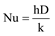(12)

where h is the convective heat transfer coefficient. The average Nusselt number is computed as:(13)

where dAs is the infinitesimal area of the wall surface. Friction factor determines the hydraulic resistance and is calculated as:(14)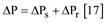(15)

where ∆P is the total pressure drop, ∆Ps is the pressure loss across the stationary pipe (N=0) and ∆Pr is the pressure loss due to rotation.

4.1. Plain Pipe

Rotation introduces coriolis and centripetal forces which yields secondary flow. The strength of the generated secondary flow affects the heat transfer rate. Variation of the average Nusselt number versus the axial Reynolds number for the plain pipe at different rotation rates are shown in Figure 4. From the graph, it is obvious that increasing rotation rate enhances the thermal performance. Nusselt number enhancement of approximately 3 % and 12% was observed at rotation rates of 1 (N=1) and 2 (N=2) respectively. At rotation rate of 3 (N=3), Nusselt number enhancement of about 25% was achieved. Nusselt number augmentation can be associated with the intensity of the secondary flow generated which increases with increasing rotation rate (N). In addition, it was recognized that increasing the axial Reynolds number also augmented the Nusselt number.

• Figure 4. Nusselt number vs. axial Reynolds number for plain pipe
• Figure 5. Pressure drop vs. axial Reynolds number for plain pipe

Figure 5 presents the distribution of the total pressure drop across the plain pipe at varying rotation rate. From the graph, it is evident that the pressure loss depends significantly on the rotation rate. Chong 17 determined the total pressure drop (∆P) across the stator-rotor gap as the sum of the pressure drop in the stationary case (∆Ps) and the pressure drop incurred due to rotation (∆Pr). Simulation results verifies that increasing the rotation rate increased the pressure drop across the rotating pipe. The maximum pressure drop was observed at rotation rate of 3 (N=3). Moreover, larger pressure losses were noticed at higher axial Reynolds numbers.

4.2. Twisted Tape Inserts

CFD results showing the axial velocity contour and vector field of the rotating pipe equipped with twisted tape are shown in Figure 6. Contours display the cross section of the velocity distribution at half the pipe length. The corresponding direction of these velocities are represented with vector fields. Simulation results showed that the inserted twisted tape divided the flow region into two halves. This caused a reduction in cross section area which led to an increase in the velocity of the flow. For all twist ratios studied, the maximum flow velocity was sited between the fluid core region and the walls of the twisted tape. These high near wall velocities contributed to the formation of extra swirl in the pipe. From Figure 6, it was observed that the strongest swirl was produced at twist ratio of 3. It was also noticed that the intensity of swirl generated at twist ratio of 5 was higher than at twist ratio of 7. Therefore, it was concluded that, decreasing the twist ratio of the inserted tape increased the flow velocity and the intensity of the swirl.

• Figure 6. Axial velocity contour for N=3.

The distribution of the average Nusselt number against the axial Reynolds numbers at different rotation rates are depicted in Figure 7. From the results, it is evident that the inserted tape enhanced the average Nusselt number of the pipe at all rotation rates. This remarkable heat transfer augmentation can be linked to increase in the strength of secondary flow due to additional swirl created by the twisted tape. Swirl causes boundary layer disturbance and enhances fluid mixing. The magnitude of swirl produced depends on the twist ratio of the tape. At rotation rate of 1 (N=1), Nusselt number enhancement of around 14 %, 17 % and 24 % was observed for twist ratio of 7, 5 and 3 respectively. The difference in Nusselt number value from twist ratio of 7 to 5 is about 2.5 % and is less than the change in Nusselt number from twist ratio of 5 to 3 which is about 6.7%. For all rotation rates studied, the inserted tape with twist ratio of 3 had the best thermal performance. This proves that decreasing twist ratio increases secondary flow intensity to enhance heat transfer.

• Figure 7. (a) Nusselt number vs. axial Reynolds Number at N=1
• Figure 7. (b) Nusselt number vs. axial Reynolds Number at N=2
• Figure 7. (c) Nusselt number vs. axial Reynolds Number at N=3

Figure 8(a), Figure 8(b) and Figure 8 (c) depicts the variation of the friction factor versus the axial Reynolds number at different rotation rate. From the results, it is observed that decreasing the twist ratio of the inserted tape increases the friction factor. At rotation rate of 3 (N=3), the maximal friction factor of the enhanced pipe (P/W=3) is about 8.5 times that of the plain pipe. It is also noted that, increasing the rotation rate increased the friction factor. Contrast with the average Nusselt number, the friction factor decreases with an increase of the axial Reynolds number.

• Figure 8. (a) Friction factor vs. axial Reynolds Number at N=1
• Figure 8. (b) Friction factor vs. axial Reynolds Number at N=2
• Figure 8. (c) Friction factor vs. axial Reynolds Number at N=3

### 5. Conclusion

In the current research, flow in an axially rotating pipe with isoflux wall condition was studied numerically using the RANS solver of ANSYS Fluent 19.2. Closure to the RANS solver was achieved with the realizable k-ε turbulent model. The computations were conducted for various rotation rates (0 ≤ N ≤ 3) and for axial Reynolds numbers between 10000 and 30000 (10000 ≤ Rea ≤ 30000). CFD results revealed that increasing the rotation rate augmented the Nusselt number. Nusselt number augmentation was linked to the strength of secondary flow generated which increases with increase of the rotation rate. Twisted tape produced extra swirl to further enhance the heat transfer rate. The intensity of swirl created by the tape depended considerably on the twist ratio. Simulation results showed that decreasing the twist ratio of the inserted tape increased the heat transfer rate.

### Acknowledgements

We acknowledge the support from NASA NNH16ZEA001N: Electric Propulsion: Challenges and Opportunities.

### References

  Weigand, B., & Beer, H. (1994). On the universality of the velocity profiles of a turbulent flow in an axially rotating pipe. Applied scientific research, 52(2), 115-132. In article View Article  Yang, W. J., Fann, S., & Kim, J. H. (1994). Heat and fluid flow inside rotating channels. In article View Article  Ould-Rouiss, M., Dries, A., & Mazouz, A. (2010). Numerical predictions of turbulent heat transfer for air flow in rotating pipe. International Journal of Heat and Fluid Flow, 31(4), 507-517. In article View Article  Davis, J., Ganju, S., Ashton, N., Bailey, S., & Brehm, C. (2019). A DNS Study to Investigate Turbulence Suppression in Rotating Pipe Flows. In AIAA Aviation 2019 Forum (p. 3639). In article View Article  NISHIBORI, K., KIKUYAMA, K., & MURAKAMI, M. (1987). Laminarization of Turbulent Flow in the Inlet Region of an Axially Rotating Pipe: Fluids Engineering. JSME International journal, 30(260), 255-262. In article View Article  Borisenko, A. I., Kostikov, O. N., & Chumachenko, V. I. (1973). Experimental investigation of turbulent flow characteristics in a rotating channel. Inzhenerno-Fizicheskii Zhurnal, 24, 1103-1108. In article  Pedley, T. J. (1968). On the instability of rapidly rotating shear flows to non-axisymmetric disturbances. Journal of Fluid Mechanics, 31(3), 603-607. In article View Article  Pedley, T. J. (1969). On the instability of viscous flow in a rapidly rotating pipe. Journal of Fluid Mechanics, 35(1), 97-115. In article View Article  Nagib, H. M., Lavan, Z., Fejer, A. A., & Wolf Jr, L. (1971). Stability of pipe flow with superposed solid body rotation. The Physics of Fluids, 14(4), 766-768. In article View Article  M. Murakami, K. Kikuyama (1980), Turbulent flow in axially rotating pipes, J. Fluids Eng. 102 (1), 97-103. In article View Article  Kikuyama, K., Murakami, M., & Nishibori, K. (1983). Development of three-dimensional turbulent boundary layer in an axially rotating pipe. Journal of Fluids Engineering, 105(2), 154-160. In article View Article  Imao, S., Itoh, M., & Harada, T. (1996). Turbulent characteristics of the flow in an axially rotating pipe. International journal of heat and fluid flow, 17(5), 444-451. In article View Article  KIKUYAMA, K., MURAKAMI, M., NISHIBORI, K., & MAEDA, K. (1983). Flow in an Axially Rotating Pipe: A calculation of flow in the saturated region. Bulletin of JSME, 26(214), 506-513. In article View Article  Bradshaw, P. (1969). The analogy between streamline curvature and buoyancy in turbulent shear flow. Journal of Fluid Mechanics, 36(1), 177-191. In article View Article  Seghir-Ouali, S., Saury, D., Harmand, S., Phillipart, O., & Laloy, D. (2006). Convective heat transfer inside a rotating cylinder with an axial air flow. International journal of thermal sciences, 45(12), 1166-1178. In article View Article  Gai, Y., Kimiabeigi, M., Widmer, J. D., Chong, Y. C., Goss, J., SanAndres, U., & Staton, D. A. (2017, May). Shaft cooling and the influence on the electromagnetic performance of traction motors. In 2017 IEEE International Electric Machines and Drives Conference (IEMDC) (pp. 1-6). IEEE. In article View Article  Chong, Y. C. (2015). Thermal analysis and air flow modelling of electrical machines. In article  Joucaviel, M., Gosselin, L., & Bello-Ochende, T. (2008). Maximum heat transfer density with rotating cylinders aligned in cross-flow. International Communications in Heat and Mass Transfer, 35(5), 557-564. In article View Article  Sun, X., & Cheng, M. (2012). Thermal analysis and cooling system design of dual mechanical port machine for wind power application. IEEE Transactions on Industrial Electronics, 60(5), 1724-1733. In article View Article  Lian, W., & Han, T. (2019). Flow and heat transfer in a rotating heat pipe with a conical condenser. International Communications in Heat and Mass Transfer, 101, 70-75 In article View Article  Yousif, A. H., & Khudhair, M. R. (2019). Enhancement Heat Transfer in a Tube Fitted with Passive Technique as Twisted Tape Insert-A Comprehensive Review. American Journal of Mechanical Engineering, 7(1), 20-34. In article  Dewan, A., Mahanta, P., Raju, K. S., & Kumar, P. S. (2004). Review of passive heat transfer augmentation techniques. Proceedings of the Institution of Mechanical Engineers, Part A: Journal of Power and Energy, 218(7), 509-527. In article View Article  Koch, R. (1960). Pressure loss and heat transfer for turbulent flow (No. AEC-TR-3875). ATOMIC ENERGY COMMISSION WASHINGTON DC. In article  Kreith, F., & Margolis, D. (1959). Heat transfer and friction in turbulent vortex flow. Applied Scientific Research, Section A, 8(1), 457-473. In article View Article  Seigel, L. G. The effect of turbulence promoters on heat transfer coefficients of water flowing in a horizontal tube. Heating, Piping and Air Conditioning, 1946, 18, 111-114. In article  Date, A. W. (1974). Prediction of fully-developed flow in a tube containing a twisted-tape. International Journal of Heat and Mass Transfer, 17(8), 845-859. In article View Article  Hong, S. W., & Bergles, A. E. (1976). Augmentation of laminar flow heat transfer in tubes by means of twisted-tape inserts. In article View Article  Wang, L., & Sunden, B. (2002). Performance comparison of some tube inserts. International Communications in Heat and Mass Transfer, 29(1), 45-56. In article View Article  Lin, Z. M., & Wang, L. B. (2009). Convective heat transfer enhancement in a circular tube using twisted tape. Journal of Heat Transfer, 131(8), 081901. In article View Article

Published with license by Science and Education Publishing, Copyright © 2020 Obed Y.W. Abotsi and J.P. KizitoThis work is licensed under a Creative Commons Attribution 4.0 International License. To view a copy of this license, visit http://creativecommons.org/licenses/by/4.0/